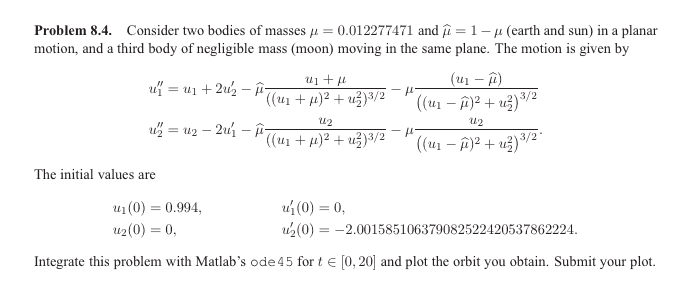# Using ODE45 on Matlab to Integrate A Problem

• ver_mathstats

#### ver_mathstats

Homework Statement
The homework statement is given below in a picture.
Relevant Equations
ODE 45 MatlabI got the answer for this question but I was wondering about stepsize when it comes to a problem like this? Is there a way to change the step size? Would my step size change in line 20, or would it change in line 3? I tried changing line 3 to be t=0:100:17.1 but then I get a error message, so would I just be changing line 20? x = linspace (0,17.1,10000); so 10 000 would be a fixed step size? I don't know how to proceed from this so any help would be appreciated.

Matlab:
mu = 0.012277471;
mu_hat = 1 - mu;
t = [0, 17.1];
u1_0 = 0.994;
u1_prime_0 = 0;
u2_0 = 0;
u2_prime_0 = -2.001585106379082522420537862224;
y_0 = [u1_0, u1_prime_0, u2_0, u2_prime_0]';
% y’ = f(y);
% y = (u1, u1’, u2, u2’)T;
Func = @(t, y)[
y(2);
y(1) + 2*y(4) - mu_hat*(y(1)+mu)/(((y(1)+mu)^2+y(3)^2)^(3/2))...
- mu*(y(1)- mu_hat)/(((y(1)-mu_hat)^2+y(3)^2)^(3/2));
y(4);
y(3) - 2*y(2) - mu_hat*y(3)/(((y(1)+mu)^2+y(3)^2)^(3/2)) - ...
mu*y(3)/(((y(1)-mu_hat)^2+y(3)^2)^(3/2))];

solution = ode45(Func, t, y_0,[10^-2,0]);
x = linspace (0,17.1,10000);
y1 = deval(solution,x,1);
y2 = deval(solution,x,3);
plot (y1,y2);
print -depsc ’odeorbit.eps’

Given that line 20 comes after the call to ode45, do you think it could affect the step size?

How do you think ode45 works out what step size to use? Do you think it uses a fixed step size? Where do you think you could find out these things?